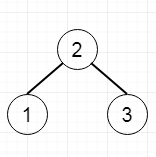# Validate Binary Search Tree in Python

Suppose we have a binary tree, determine to check whether it is a valid binary search tree (BST) or not. Assume a BST is defined as follows –

• The left subtree of a node holds only nodes with keys smaller than the node's key.
• The right subtree of a node holds only nodes with keys larger than the node's key.
• Both the left and right subtrees must also be binary search trees.

So if the tree is like –The output will be true.

To solve this, we will follow these steps –

• Create one recursive function called solve(), this will take root, min and max, the method will be like
• if root is null, then return true
• if value of root <= min or value of root >= max, then return false
• return the (solve(left of root, min, root value) AND solve(right of root, root value, max))
• call the solve() method initially, by passing root, and – inf as min and inf as max.

## Example(Python)

Let us see the following implementation to get a better understanding −

Live Demo

class TreeNode:
def __init__(self, data, left = None, right = None):
self.data = data
self.left = left
self.right = right
def insert(temp,data):
que = []
que.append(temp)
while (len(que)):
temp = que
que.pop(0)
if (not temp.left):
if data is not None:
temp.left = TreeNode(data)
else:
temp.left = TreeNode(0)
break
else:
que.append(temp.left)
if (not temp.right):
if data is not None:
temp.right = TreeNode(data)
else:
temp.right = TreeNode(0)
break
else:
que.append(temp.right)
def make_tree(elements):
Tree = TreeNode(elements)
for element in elements[1:]:
insert(Tree, element)
return Tree
class Solution(object):
def isValidBST(self, root):
return self.solve(root,-1000000000000000000000,1000000000000000000000)
def solve(self,root,min_val,max_val):
if root == None or root.data == 0:
return True
if (root.data <= min_val or root.data >=max_val):
return False
return self.solve(root.left,min_val,root.data) and self.solve(root.right,root.data,max_val)
ob1 = Solution()
tree = make_tree([3,1,4,None,2,None,5])
print(ob1.isValidBST(tree))
tree = make_tree([5,1,4,None,None,3,6])
print(ob1.isValidBST(tree))

## Input

[3,1,4,null,2,null,5]
[5,1,4,null,null,3,6]

## Output

true
false